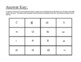digital

# Solving Systems of Equations: 4 x 4 PuzzleSubject
Resource Type
Formats

TpT Digital Activity

PDF (257 KB|5 pages)
Standards
TpT Digital Activity
Add notes & annotations through an interactive layer and assign to students via Google Classroom.
Also included in
1. This bundle contains 10 engaging, more than just a worksheet activities that I use to help my students learn about solving systems of equations. The different types of activities that are included in this bundle are1) Introduction to Systems of Equations by Exploring Real World Scenarios ResourceStu
\$15.99
\$24.50
Save \$8.51
2. This bundle contains my most engaging resources and activities that I use to help my students master the 8th grade common core standards.  Individually, this bundle would cost \$193. SAVE \$93 dollars by buying this bundle! The different types of activities that are included in this bundle are1) Dif
\$100.00
\$193.00
Save \$93.00
• Product Description
• Standards

Students practice solving systems of equations by putting together a math puzzle! Students are able to solve these problems primarily by the elimination and substitution techniques.

This is great for group work or for centers and can be a great formative assessment for teachers.

Real World: Solving Systems of Equations Word Problems

Math Murder Mystery! Solving Systems of Equations

Solving Systems of Equations Differentiated Math Mazes

Solving Systems of Equations Scavenger Hunt

Solving Systems of Equations Error Analysis

Solving Systems of Equations Jeopardy Game

Sum It Up! Differentiated Solving Systems of Equations Activity

Solving Systems of Equations Task Cards

Solving System of Equations Anchor Charts

Adding and Subtracting Integers: 4 x 4 Puzzle

Multiplying and Dividing Integers: 4 x 4 Puzzle

Converting Fractions to Decimals: 4 x 4 Puzzle

Approximating Square Roots: 4 x 4 Puzzle

Solving One Step Equations: 4 x 4 Puzzle

Solving Two Step Equations: 4 x 4 Math Puzzle

Simplifying Algebraic Expressions: 4 x 4 Math Puzzle

Evaluating Algebraic Expressions: 4 x 4 Puzzle

Solving Proportions Using Cross Products: Math Puzzle

Solving Multi-Step Inequalities: 4 x 4 Puzzle

Calculating Slope From Two Points: 4 x 4 Puzzle

Finding the Midpoint: 4 x 4 Puzzle

Solving Absolute Value Equations: 4 x 4 Puzzle

Exponential Properties: 4 x 4 Puzzle

Factoring and FOILING Polynomials: 4 x 4 Puzzle

Solving Radical Equations: 4 x 4 Puzzle

Calculating Mean, Median, and Mode: 4 x 4 Puzzle

**Finally, don't forget to rate and comment!**

And if you like this math puzzle be sure to follow my store! More FREE math puzzles are coming soon!

to see state-specific standards (only available in the US).
Solve systems of two linear equations in two variables algebraically, and estimate solutions by graphing the equations. Solve simple cases by inspection. For example, 3𝘹 + 2𝘺 = 5 and 3𝘹 + 2𝘺 = 6 have no solution because 3𝘹 + 2𝘺 cannot simultaneously be 5 and 6.
Understand that solutions to a system of two linear equations in two variables correspond to points of intersection of their graphs, because points of intersection satisfy both equations simultaneously.
Analyze and solve pairs of simultaneous linear equations.
Total Pages
5 pages
Included
Teaching Duration
30 minutes
Report this Resource to TpT
Reported resources will be reviewed by our team. Report this resource to let us know if this resource violates TpT’s content guidelines.
• Ratings & Reviews
• Q & A

Teachers Pay Teachers is an online marketplace where teachers buy and sell original educational materials.